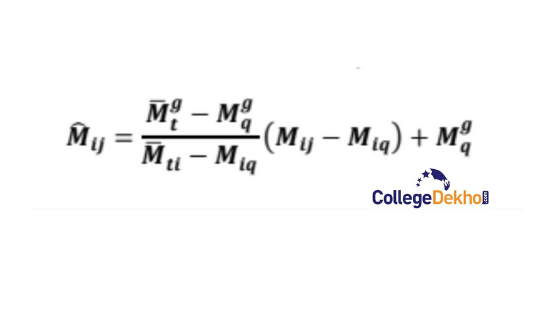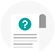Become Job Ready with CollegeDekho Assured Program

# GATE 2023 Normalisation Process: How to Calculate GATE Score

GATE 2023 result has been released after the GATE 2023 exam. This article will cover the overview of the GATE 2023 normalisation process which will demonstrate why is it done, and how the final score of GATE is calculated using the normalisation process.How Scores Are Calculated for the GATE Exam - The Graduate Aptitude Test in Engineering (GATE) 2023 will be conducted in multiple sessions and time slots for 29 subjects. Some GATE courses, such as mechanical, computer science, civil engineering, and electrical, will be evaluated in more than one session.GATE is one of the most popular entrance exams in India to get admission into various postgraduate programs in IITs, NITs, IIITs, and PSU jobs. The GATE 2023 normalisation technique is used to generate an unbiased score because the level of difficulty of the same paper conducted in two slots may differ. To determine a score that is equal to the level of difficulty, the exam authorities will employ a normalisation procedure. The candidates' score out of 1000 will subsequently be determined using the normalised score from the GATE 2023 exam. The GATE score of a candidate is the number of points earned out of a possible 1000. Students frequently question how the GATE is normalised and how scores are determined. To learn more about GATE normalisation and GATE score calculation, continue reading.

## About GATE Normalisation Process 2023

GATE 2022 papers will be conducted in multiple sessions. Different sessions will have different levels of difficulty for the GATE paper. Hence, in order to conduct a fair examination and evaluate a fair cutoff score, the normalisation process will be done. Often candidates who have appeared for the exam get confused as to how the normalisation process is done and how is the final score calculated for GATE. This article will demonstrate the formula and process for the GATE normalisation process to help students get an idea about the same.

 Analysis of GATE Rank vs Marks vs Score GATE Cutoff

## Difference Between GATE Score and Normalised Score

The GATE score and the normalised score are not the same and are calculated using different formulas and processes. Below given are some of the points which demonstrate how the GATE score differs from the normalised score:

• While the GATE score is done for all the papers and is reflected on the scorecard of GATE, the normalised score is only calculated for the papers conducted in multiple sessions and are reflected through the GATE result.

• The examination board does not use the normalised score to calculate the final marks of the candidate. The GATE score is used to calculate the final marks.

• While all the candidates who have appeared for GATE 2022 can avail of the normalised score, only the qualified students can avail of the GATE score.

The below section will demonstrate the detailed process of the GATE normalisation process, along with the formula used to derive the normalised score for GATE.

## The Formula for GATE Normalisation Process

The standard deviation and variance formula is used for calculating the normalised score for the candidates appearing in the multi-session papers of GATE.

The actual formula which is used to calculate the GATE normalisation score is:Where,

mij: the marks obtained by the candidate in the session

mgt: the average marks of candidates for all sessions

mgq: the sum of mean & Standard deviation for all sessions

mti: the average marks of candidates for one session

miq: the sum of mean & Standard deviation for one session

Let us understand the normalisation process with the help of a case study.

Let us assume there are 5 candidates who have appeared for the GATE multi-session papers. The marks obtained by all of the 5 candidates out of full marks are as follows:

 Candidate Marks Obtained C1 144 C2 152 C3 142 C4 132 C5 120

Now, the average score for the marks obtained by all the candidates will be calculated using the formula:

C1 + C2 + C3 + C4 + C5/ 5

Which will be: 144 + 152 + 142 + 132 + 120/ 5

= 690/ 5

=183, which is the average score

Further, the variance of the average score for all the candidates will be calculated using the formula:

Square of the sum of (Actual score- Average score)/ total candidates

Which will be: sq. [(144-138) + (152-138) - (142-138) - (138-132) + (138-120)]/ 5

Variance will be: sq. 6 + sq. 14 + sq. 4 +sq. 6 + sq. 18/ 5

= 24

Now, the standard deviation for the calculated variance will be derived using the formula: Sq. root (variance)

Which will be: sq. root (24)

= 4.89

= 5 (Nearest sum)

This will be the final normalised score.

After the calculation of the normalised score, the final score is released by the GATE examination authority for all the papers.

## GATE 2023 Score Card

After the GATE exam, the marks obtained by the candidate in the papers will be released. The date for the declaration of GATE 2023 result  will be announced soon after the exam is conducted. Finally, after the normalisation score, the scorecard will be released for the qualifying candidates on 21st March. The GATE 2022 Scorecard is really important as all the admission for the candidates will be done on the basis of the marks in the scorecard of the candidate. Students are advised to visit the official website to download the GATE scorecard as per the prescribed date. The scorecard for GATE will consist of the following details:

• The score obtained by the candidate in the GATE 2022 exam.

• Validity of Score

• All India Rank for the candidate for that paper

• Total marks out of 100

• GATE 2022 Qualifying marks

• The number of candidates who appeared for the exam.

Related Articles

 Exam Analysis for GATE MT Recruitment in PSU without GATE score Exam Analysis for GATE PE Exam Analysis for GATE Physics GATE low score: List of accepting colleges Get all the exam instructions and tips for the GATE 2022 exam GATE cutoff for IIT Bombay GATE cutoff for IIT Delhi GATE cutoff for IIT Kharagpur GATE cutoff for IIT Kanpur GATE cutoff for IIT Gandhinagar GATE cutoff for IIT Ropar GATE cutoff for IIT Madras GATE cutoff for IIT Bhubaneswar GATE cutoff for IIT Patna GATE cutoff for IIT Indore

For more details related to exams, stay updated to Collegedekho.

### GATE Previous Year Question PaperGATE Production and Industrial Engineering (PI) Question Paper 2019GATE Production and Industrial Engineering (PI) Answerkey 2019GATE Physics (PH) 2019GATE Petroleum Engineering (PE) 2019GATE Petroleum Engineering (PE) Answer key 2019GATE Mining Engineering (MN) 2019GATE Metallurgical Engineering (MT) Answer key 2019GATE Mechanical Engineering (ME1) 2019GATE Mechanical Engineering (ME02) Question Paper 2019GATE Mechanical Engineering (ME02) Answer key 2019GATEGATE Mathematics (MA) Answer key 2019GATE Mathematics (MA) Answer key 2019GATE Life Sciences (XL-P, Q, R, S, T, U) Question Paper 2019GATE Instrumentation Engineering (IN) 2019GATE Instrumentation Engineering (IN) Answer key 2019GATE Geology and Geophysics (GG) Question Paper 2019GATE Engineering Sciences (XE-A, B, C, D, E, F, G, H) 2019GATE Engineering Sciences (XE-A, B, C, D, E, F, G, H) Answer keys 2019GATE Electronics and Communication Engineering (EC) 2019GATE Electronics and Communication Engineering (EC) Answer key 2019Electrical Engineering 2019Gate Electrical Engg. Answerkey 2019GATE Ecology and Evolution (EY) 2019GATE Ecology and Evolution (EY) Answer key 2019GATE Computer Science and Information Technology (CS) 2019GATE Computer Science and Information Technology (CS) Answer key 2019GATE Civil Engineering (CE1) 2019GATE Civil Engineering (CE1) Answer key 2019GATE Civil Engineering (CE2) 2019GATE Chemistry (CY) 2019GATE Chemistry (CY) Answer key 2019GATE Chemical Engineering (CH) 2019GATE Chemical Engineering (CH) Answer key 2019GATE Biotechnology (BT) 2019GATE Biotechnology (BT) Answerkey 2019GATE Architecture and Planning (AR)2019GATE Architecture and Planning (AR) Answer key 2019GATE Agricultural Engineering (AG) 2019GATE Agricultural Engineering (AG) Answer key 2018GATE Agricultural Engineering (AG) Answer key 2019GATE Aerospace Engineering (AE) 2019GATE Aerospace Engineering (AE) Answer key 2019GATE 2017 AE Question PaperGTE IN 2017 question paperGATE IN 2017 Question Paper

/articles/gate-normalisation-process-how-to-calculate-gate-score/

## Related Questions

### Engineering 4th year student eligible for gate exam?

-AnonymousUpdated on September 09, 2022 11:59 AM
Diksha Sharma, Student / Alumni

Dear Student,

Yes, the students who are in their final year of graduation or are awaiting their results can also apply for GATE Exam. However, it is important for the candidates to generate their qualification degree at the time of admission. Recently, the examination authority has made several changes to the GATE eligibility criteria. To learn about the new GATE eligibility, read GATE Eligibility Requirements.

Meanwhile, you can also go through other articles related to GATE to learn more:

What’s New in GATE 2021?

GATE 2021: List of Top 25 Government & Private Colleges for M.Tech Admission

Documents Required …

### Do you have a question? Ask us.

• Typical response between 24-48 hours

• Get personalized response

• Free of Cost